# AP Calculus AB Practice Test 33

### Test Information9 questions18 minutes

Calculator Disallowed

1. Over the interval 0 ≤ x ≤ 10, the average value of the function f shown below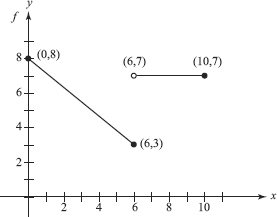2. If f (x) = 2f (x) and f (2) = 1, then f (x) =

3. The table below shows values of f (x) for various values of x:

 x -1 0 1 2 3 f ″(x) -4 -1 2 5 8

The function f could be

4. The curve x3 + x tan y = 27 passes through (3,0). Use the tangent line there to estimate the value of y at x = 3.1. The value is

5. At what value of h is the rate of increase of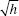twice the rate of increase of h ?

6.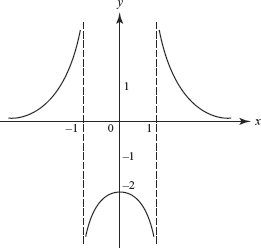The graph of a function y = f (x) is shown above. Which is true?

7. A function f (x) equals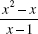for all x except x = 1. For the function to be continuous at x = 1, the value of f (1) must be

8. The number of inflection points on the graph of f (x) = 3x5 - 10x3 is

9. Suppose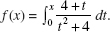It follows that